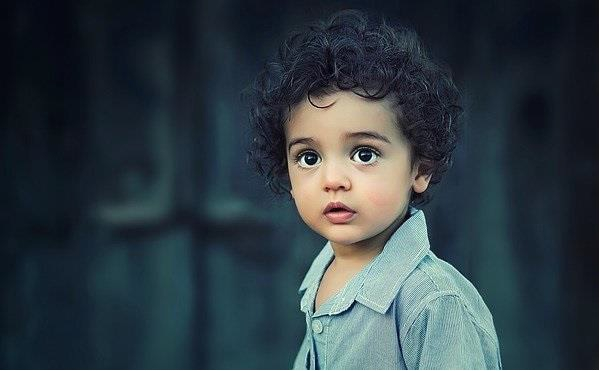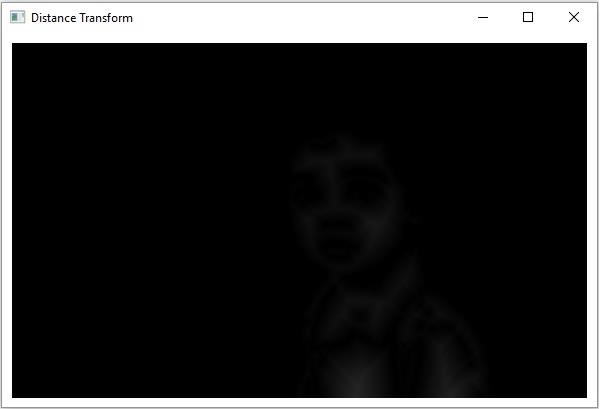# Explain OpenCV Distance Transformation using java Example

The distance transform, in general, is a derived representation of a digital image. In this operation, the gray level intensities of the points inside the foreground regions are changed to distance their respective distances from the closest 0 value (boundary).

The distanceTransform() method of the Imgproc class applies Distance Transform on the given image, this method accepts −

• Two Mat objects representing the source and destination images.

• An integer variable representing the type of the distance transformation to be applied.

• An integer value representing the mask size to be used.

## Example

import java.awt.Image;
import java.awt.image.BufferedImage;
import java.io.IOException;
import javafx.application.Application;
import javafx.embed.swing.SwingFXUtils;
import javafx.scene.Group;
import javafx.scene.Scene;
import javafx.scene.image.ImageView;
import javafx.scene.image.WritableImage;
import javafx.stage.Stage;
import org.opencv.core.Core;
import org.opencv.core.Mat;
import org.opencv.highgui.HighGui;
import org.opencv.imgcodecs.Imgcodecs;
import org.opencv.imgproc.Imgproc;
public class DistanceTransform extends Application {
public void start(Stage stage) throws IOException {
//Reading the Image from the file and storing it in to a Matrix object
String file ="D://images//boy.jpg";
//Creating an empty matrix to store the results
Mat dst = new Mat();
Mat binary = new Mat();
//Converting the gray scale image to binary image
Imgproc.threshold(src, binary, 100, 255, Imgproc.THRESH_BINARY);
//Applying distance transform
Imgproc.distanceTransform(binary, dst, Imgproc.DIST_C, 3);
Core.convertScaleAbs( dst, dst );
//Converting matrix to JavaFX writable image
Image img = HighGui.toBufferedImage(dst);
WritableImage writableImage= SwingFXUtils.toFXImage((BufferedImage) img, null);
//Setting the image view
ImageView imageView = new ImageView(writableImage);
imageView.setX(10);
imageView.setY(10);
imageView.setFitWidth(575);
imageView.setPreserveRatio(true);
//Setting the Scene object
Group root = new Group(imageView);
Scene scene = new Scene(root, 595, 400);
stage.setTitle("Distance Transform");
stage.setScene(scene);
stage.show();
}
public static void main(String args[]) {
launch(args);
}
}

## Input Image## Output

On executing, the above program generates the following windows −Updated on: 13-Apr-2020

143 Views